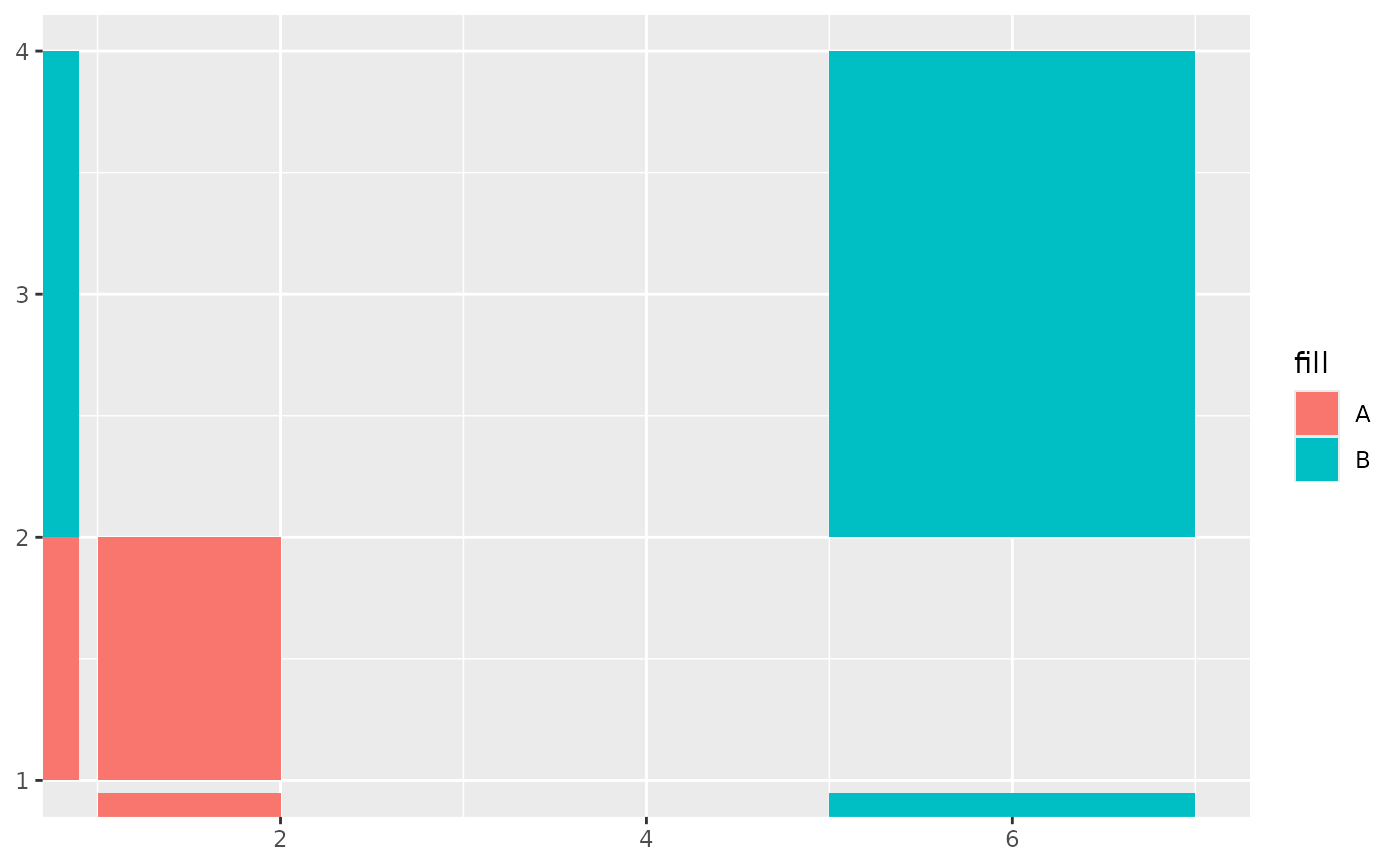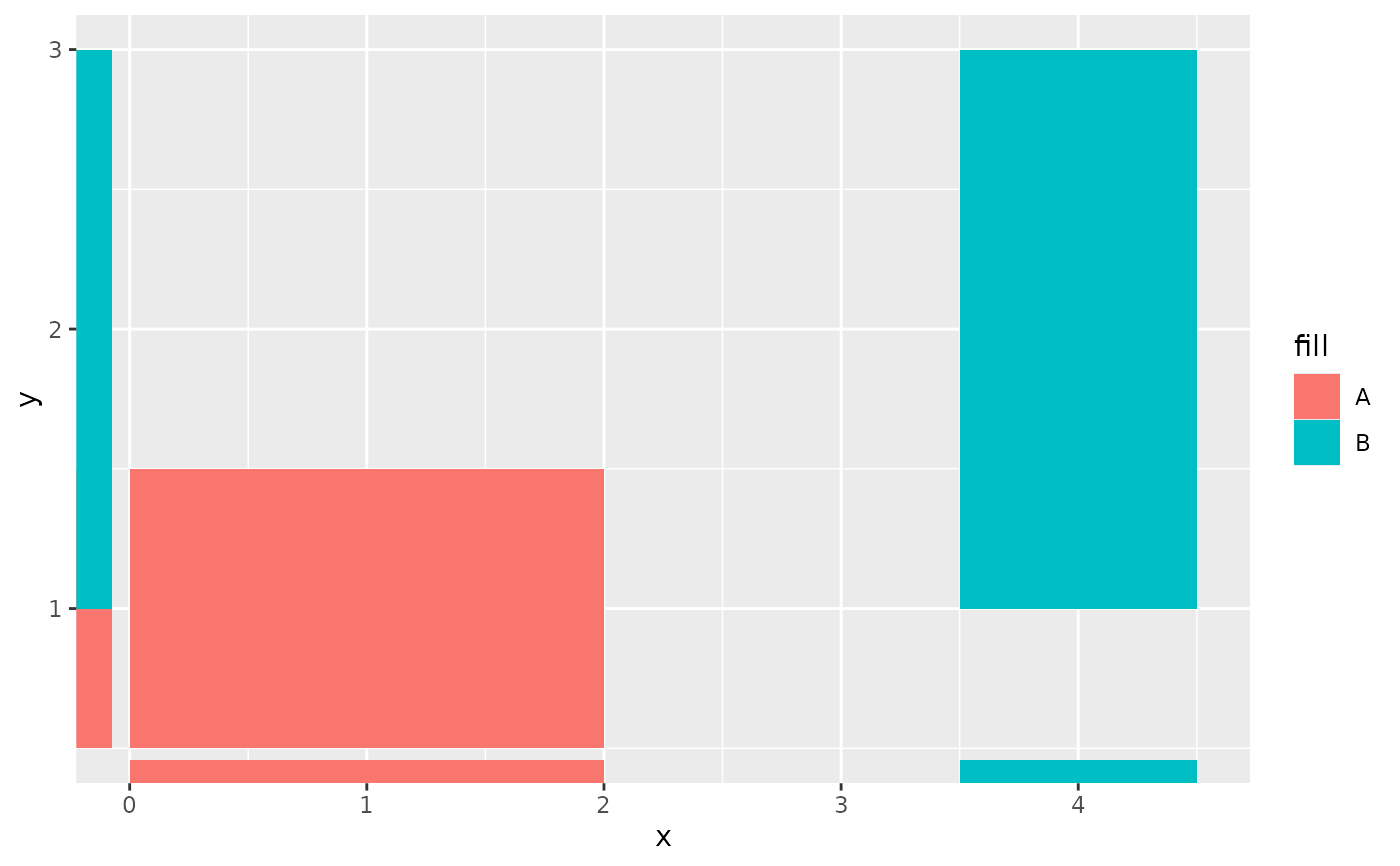Like rug plots display data points of a 2D plot as lines in the margins, this function plots rectangles in the margins. Rectangular rugs are convenient for displaying one-dimensional, ranged annotations for two-dimensional plots.

## Usage

geom_rectmargin(
mapping = NULL,
data = NULL,
stat = "identity",
position = "identity",
...,
outside = FALSE,
sides = "bl",
length = unit(0.03, "npc"),
linejoin = "mitre",
na.rm = FALSE,
show.legend = NA,
inherit.aes = TRUE
)

geom_tilemargin(
mapping = NULL,
data = NULL,
stat = "identity",
position = "identity",
...,
outside = FALSE,
sides = "bl",
length = unit(0.03, "npc"),
linejoin = "mitre",
na.rm = FALSE,
show.legend = NA,
inherit.aes = TRUE
)

## Arguments

mapping

Set of aesthetic mappings created by aes(). If specified and inherit.aes = TRUE (the default), it is combined with the default mapping at the top level of the plot. You must supply mapping if there is no plot mapping.

data

The data to be displayed in this layer. There are three options:

If NULL, the default, the data is inherited from the plot data as specified in the call to ggplot().

A data.frame, or other object, will override the plot data. All objects will be fortified to produce a data frame. See fortify() for which variables will be created.

A function will be called with a single argument, the plot data. The return value must be a data.frame, and will be used as the layer data. A function can be created from a formula (e.g. ~ head(.x, 10)).

stat

The statistical transformation to use on the data for this layer, either as a ggproto Geom subclass or as a string naming the stat stripped of the stat_ prefix (e.g. "count" rather than "stat_count")

position

Position adjustment, either as a string naming the adjustment (e.g. "jitter" to use position_jitter), or the result of a call to a position adjustment function. Use the latter if you need to change the settings of the adjustment.

...

Other arguments passed on to layer(). These are often aesthetics, used to set an aesthetic to a fixed value, like colour = "red" or size = 3. They may also be parameters to the paired geom/stat.

outside

logical of length 1 that controls whether to move the rectangles outside of the plot area. For the best results, it is probably best to set coord_cartesian(clip = "off") and avoid overlap with the default axes by changing the sides argument to "tr".

sides

A string of length 1 that controls which sides of the plot the rug-rectangles appear on. A string containing any letters in "trbl" will set it to top, right, bottom and left respectively.

length

A grid::unit() object that sets the width and height of the rectangles in the x- and y-directions respectively. Note that scale expansion can affect the look of this.

linejoin

Line join style (round, mitre, bevel).

na.rm

If FALSE, the default, missing values are removed with a warning. If TRUE, missing values are silently removed.

show.legend

logical. Should this layer be included in the legends? NA, the default, includes if any aesthetics are mapped. FALSE never includes, and TRUE always includes. It can also be a named logical vector to finely select the aesthetics to display.

inherit.aes

If FALSE, overrides the default aesthetics, rather than combining with them. This is most useful for helper functions that define both data and aesthetics and shouldn't inherit behaviour from the default plot specification, e.g. borders().

## Value

A Layer ggproto object.

## Details

By default, scales are expanded 5\ whereas the rug rectangles will occupy 3\ default. The geom_rectmargin() and geom_tilemargin() versions do the same thing, but are parametrised differently; see geom_rect().

These functions do not have hard-coded required aesthetics, since the x and y directions can be omitted by not choosing a side in the corresponding direction, i.e. y-direction variables are omitted when plotting the rug only on the top and/or bottom. This can result in errors when the aesthetics are not specified appropriately, so some caution is advised.

## Aesthetics

geom_rectmargin() requires either one of the following sets of aesthetics, but also can use both:

• xmin

• xmax

and/or:

• ymin

• ymax

geom_tilemargin() requires either one of the following sets of aesthetics, but can also use both:

• x

• width

and/or:

• y

• height

Furthermore, geom_rectmargin() and geom_tilemargin() also understand these shared aesthetics:

• alpha

• colour

• fill

• group

• linetype

• size

ggplot2::geom_rug(), geom_rect(), ggplot2::geom_tile()

## Examples

# geom_rectmargin() is parameterised by the four corners
df <- data.frame(
xmin = c(1, 5),
xmax = c(2, 7),
ymin = c(1, 2),
ymax = c(2, 4),
fill = c("A", "B")
)

ggplot(df, aes(xmin = xmin, xmax = xmax,
ymin = ymin, ymax = ymax,
fill = fill)) +
geom_rect() +
geom_rectmargin()# geom_tilemargin() is parameterised by center and size
df <- data.frame(
x = c(1, 4),
y = c(1, 2),
width = c(2, 1),
height = c(1, 2),
fill = c("A", "B")
)

ggplot(df, aes(x, y,
width = width, height = height,
fill = fill)) +
geom_tile() +
geom_tilemargin()